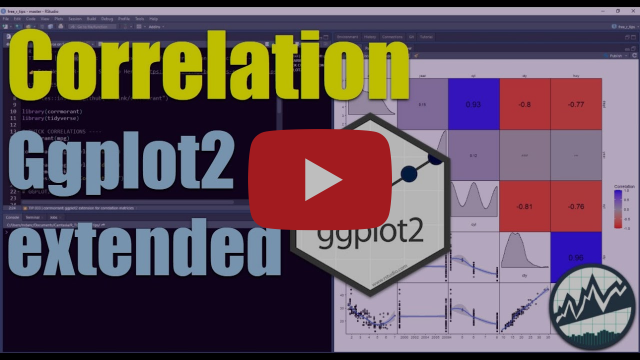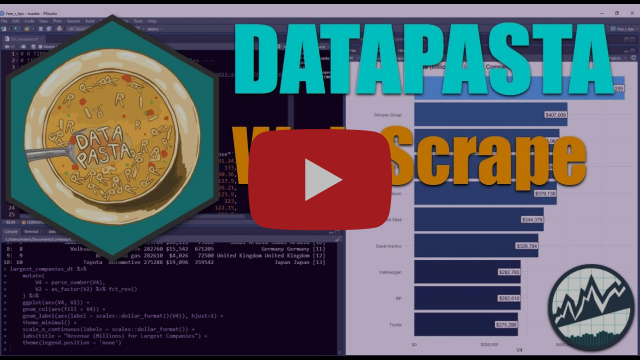### Introducing Iterative (Nested) Forecasting with Modeltime

August 25, 2021 |

Iteratively forecast with tidyverse nested modeling Why is nested forecasting important? For starters, the ability to iteratively forecast time series with many models that are trained on many individual groups has been a huge request from student...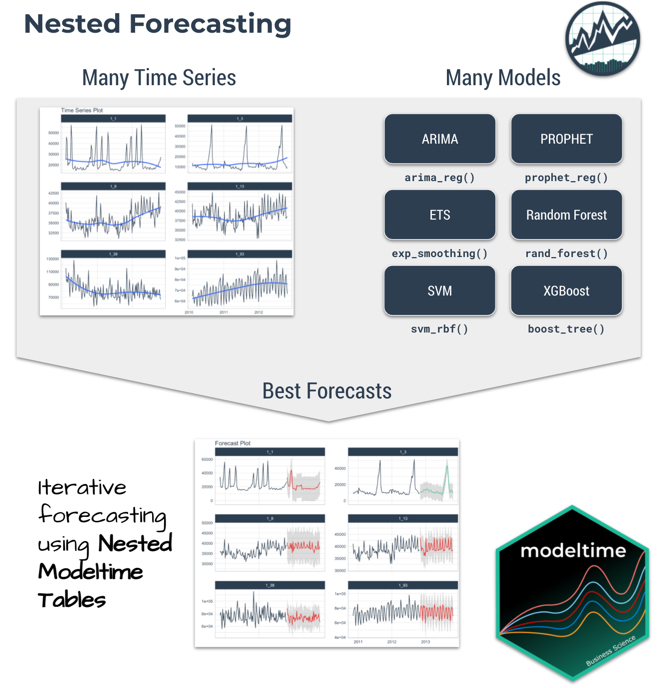### ggalt: Make a Lollipop Plot to Compare Categories in ggplot2

August 23, 2021 |

A Lollipop Plot shows the relationship between categories using a dot and a line that connects to a baseline (similar to a Bar Plot). In this short tutorial, we use ggalt to create a Lollipop Plot with the geom_lollipop() function. R-Tips Weekly This...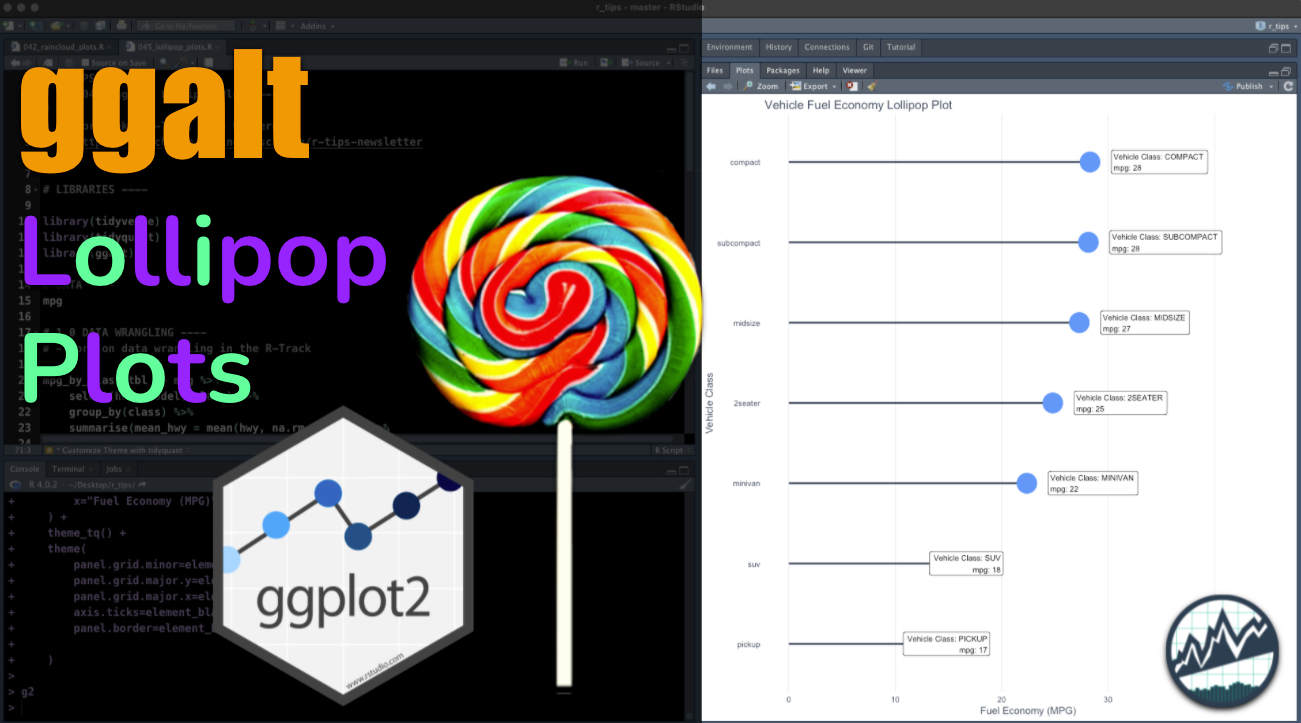### ggalt: Make a Dumbbell Plot to Visualize Change in ggplot2

August 11, 2021 |

ggalt is a ggplot2 extension that adds many new ggplot geometries. In this tutorial, we’ll learn how to make dumbbell plots for visualizing change within our data using geom_dumbbell(). R-Tips Weekly This article is part of R-Tips Weekly, a weekly vi...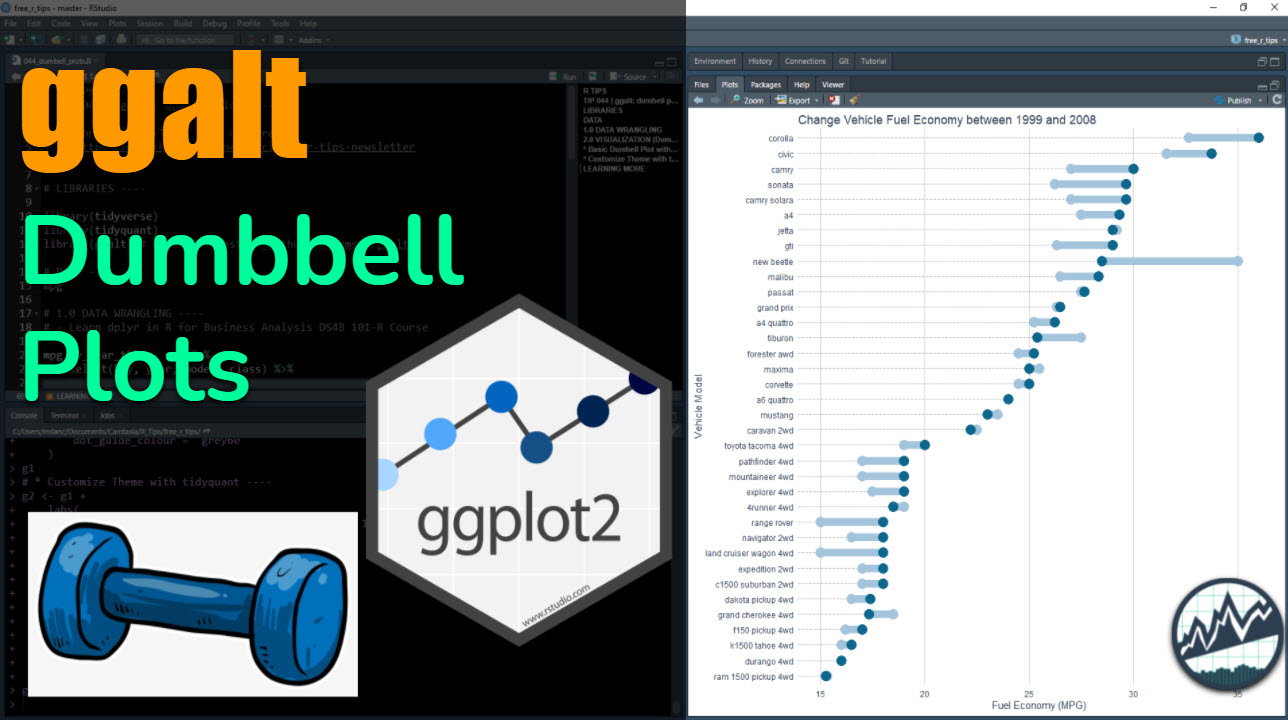### ggforce: Make a Hull Plot to Visualize Clusters in ggplot2

July 26, 2021 |

The ggforce package is a ggplot2 extension that adds many exploratory data analysis features. In this tutorial, we’ll learn how to make hull plots for visualizing clusters or groups within our data. R-Tips Weekly This article is part of R-Tips Weekly...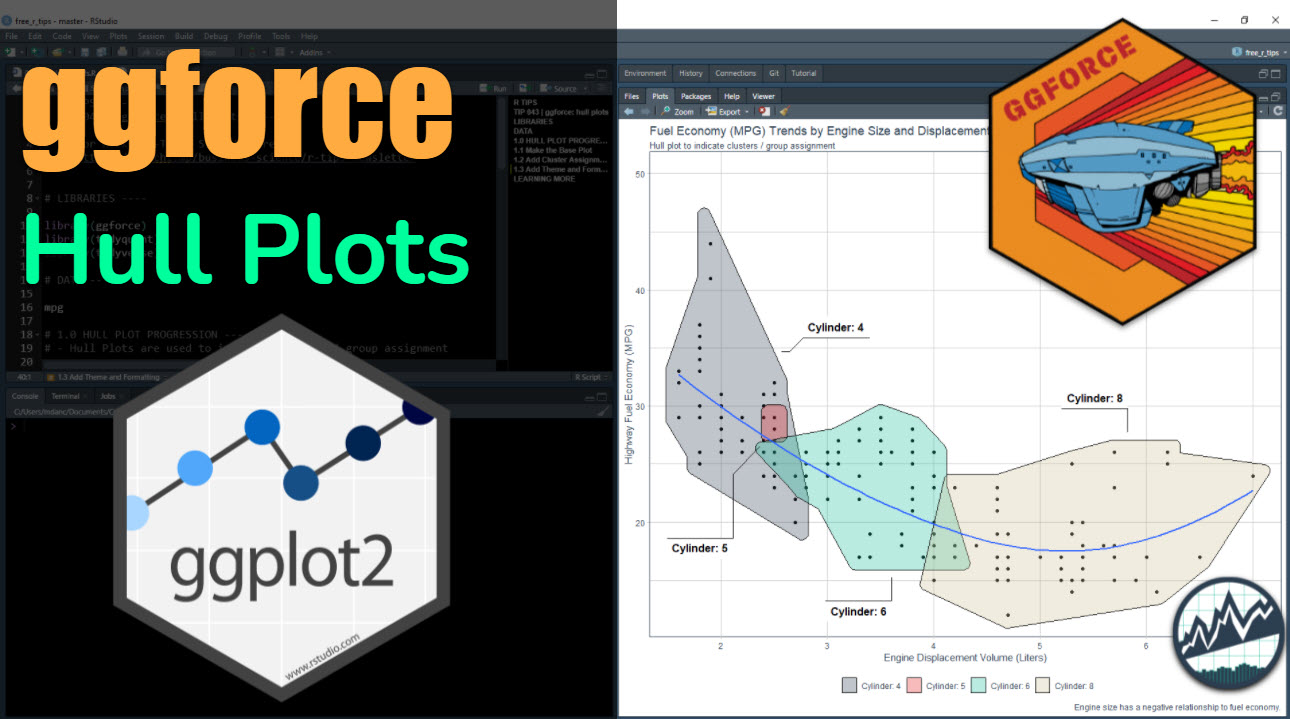### ggdist: Make a Raincloud Plot to Visualize Distribution in ggplot2

July 21, 2021 |

The ggdist package is a ggplot2 extension that is made for visualizing distributions and uncertainty. We’ll show see how ggdist can be used to make a raincloud plot. R-Tips Weekly This article is part of R-Tips Weekly, a weekly video tutorial that sh...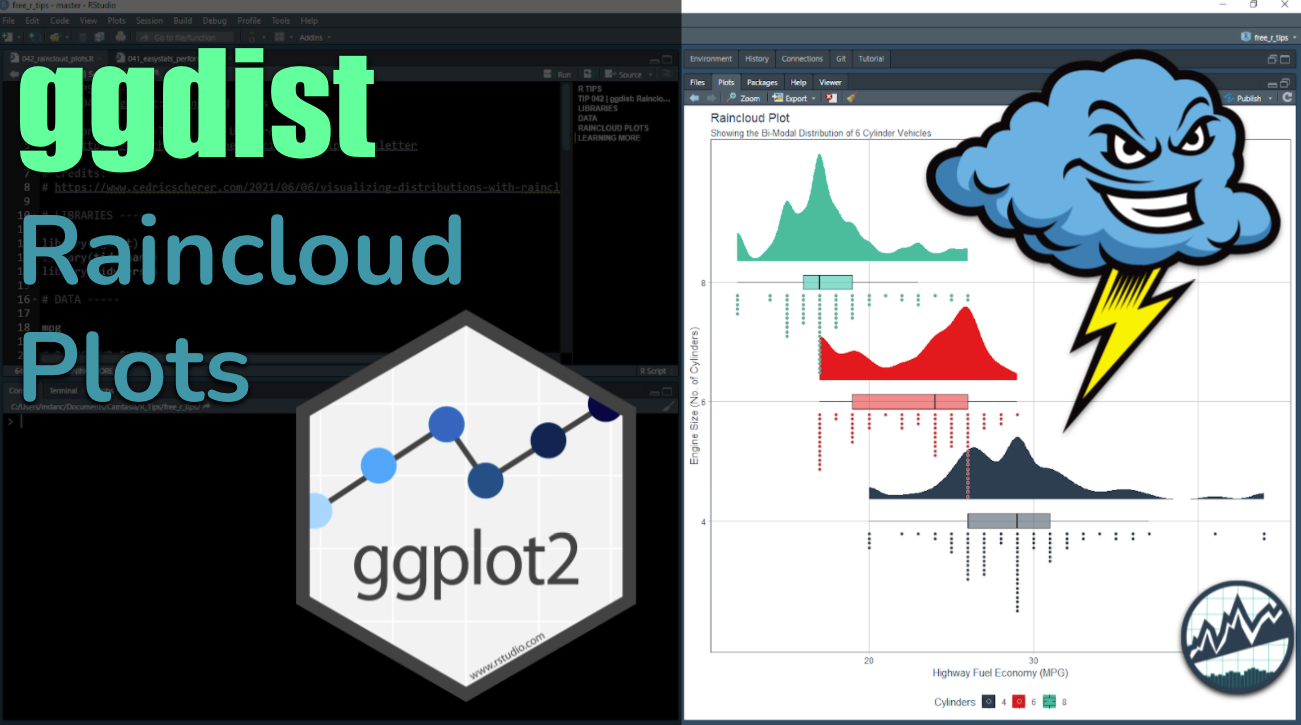### Forecasting Many Time Series (Using NO For-Loops)

July 18, 2021 |

Spending too much time on making iterative forecasts? I’m super excited to introduce the new panel data forecasting functionality in modeltime. It’s perfect for forecasting many time series at once without for-loops saving you time ⏱️ and aggravation 😞. Just say NO to for-loops for forecasting. Fitting many time series ...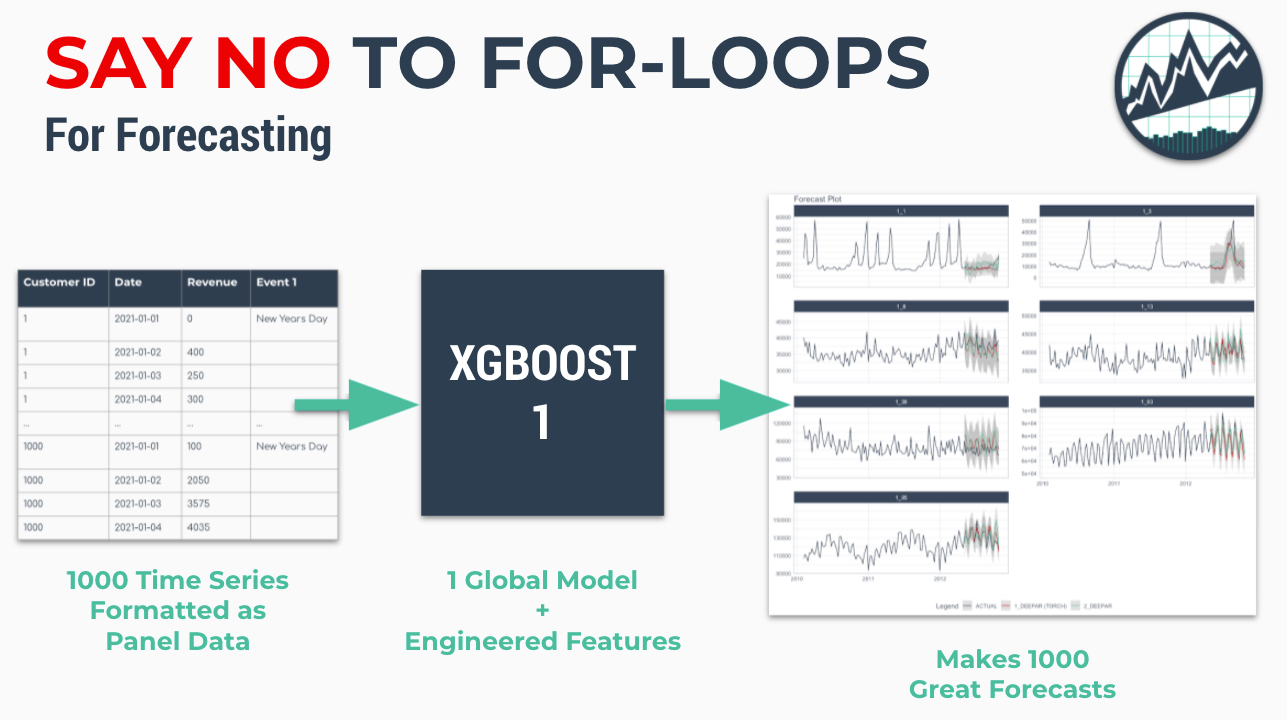### easystats: Quickly investigate model performance

July 13, 2021 |

Easystats performance is an R package that makes it easy to investigate the relevant assumptions for regression models. Simply use the check_model() function to produce a visualization that combines 6 tests for model performance. We’ll quickly: Lea...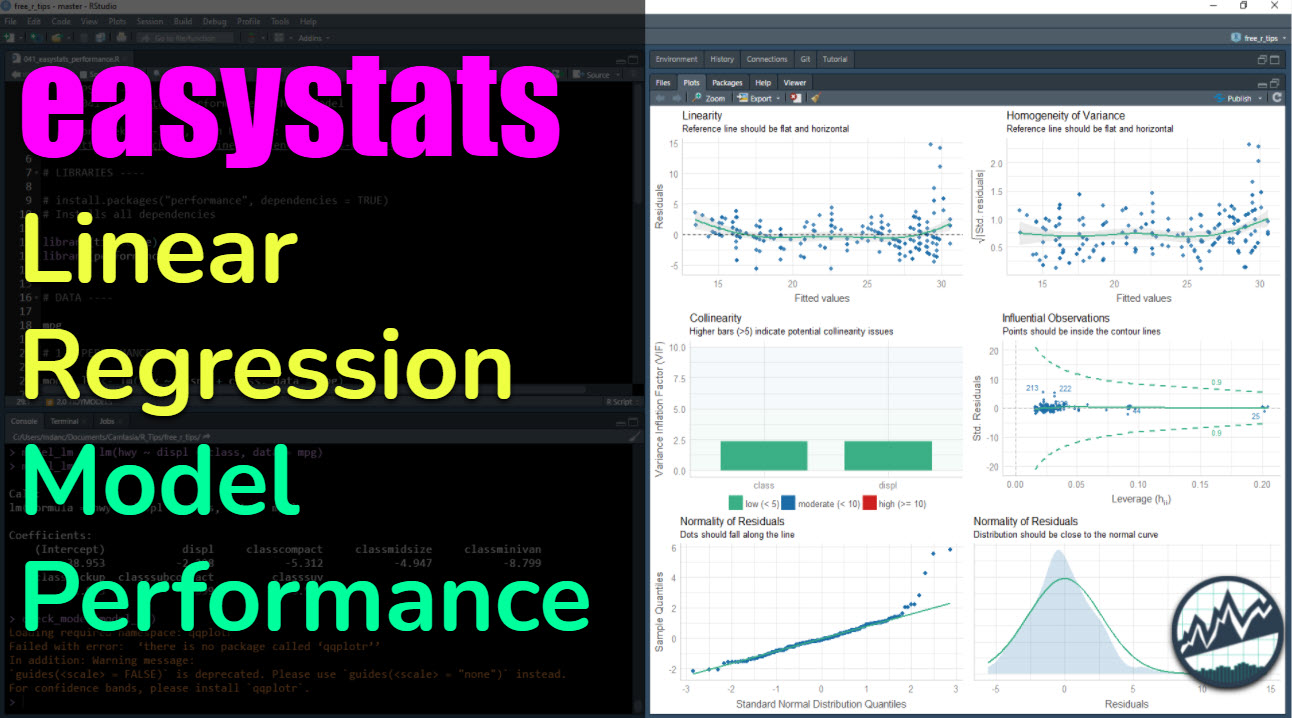### R is for Research, Python is for Production

July 11, 2021 |

👉 Sign Up For More Blog Articles 👈 Updated July 2021 Both R and Python are great. We’ll showcase some of the strengths of each language in this article by showcasing where the major development efforts are within each ecosystem. ...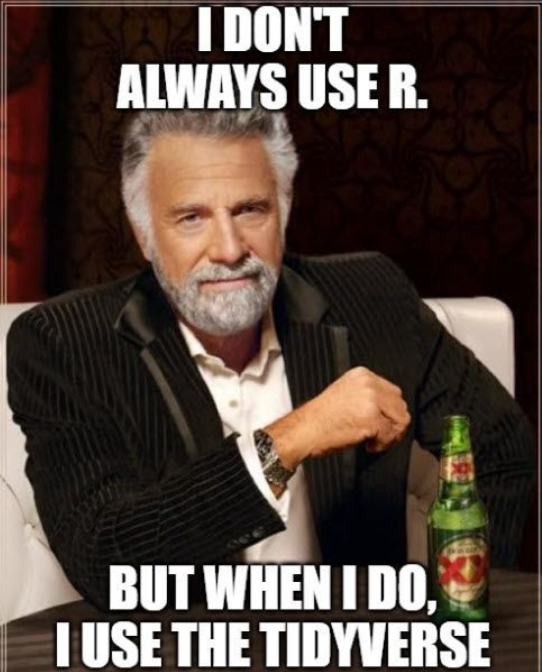### Gentle Introduction to Forecasting with Modeltime [Video Tutorial]

June 29, 2021 |

A gentle introduction to our forecasting package, modeltime. Modeltime extends the Tidymodels ecosystem for time series forecasting. Learn how to forecast with ARIMA, Prophet, and linear regression time series models in this short video tutorial. ...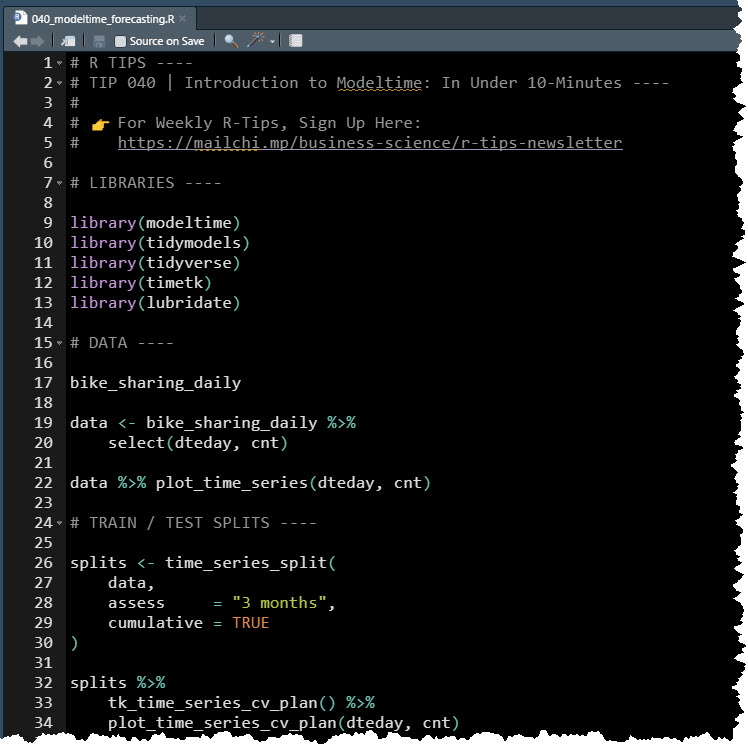### plotnine: Make great-looking correlation plots in Python

June 21, 2021 |

The plotnine library is a powerful python visualization library based on R’s ggplot2 package. In this tutorial, we show you how to make a great-looking correlation plot using pandas and plotnine. This article is part of Python-Tips Weekly, a bi-we...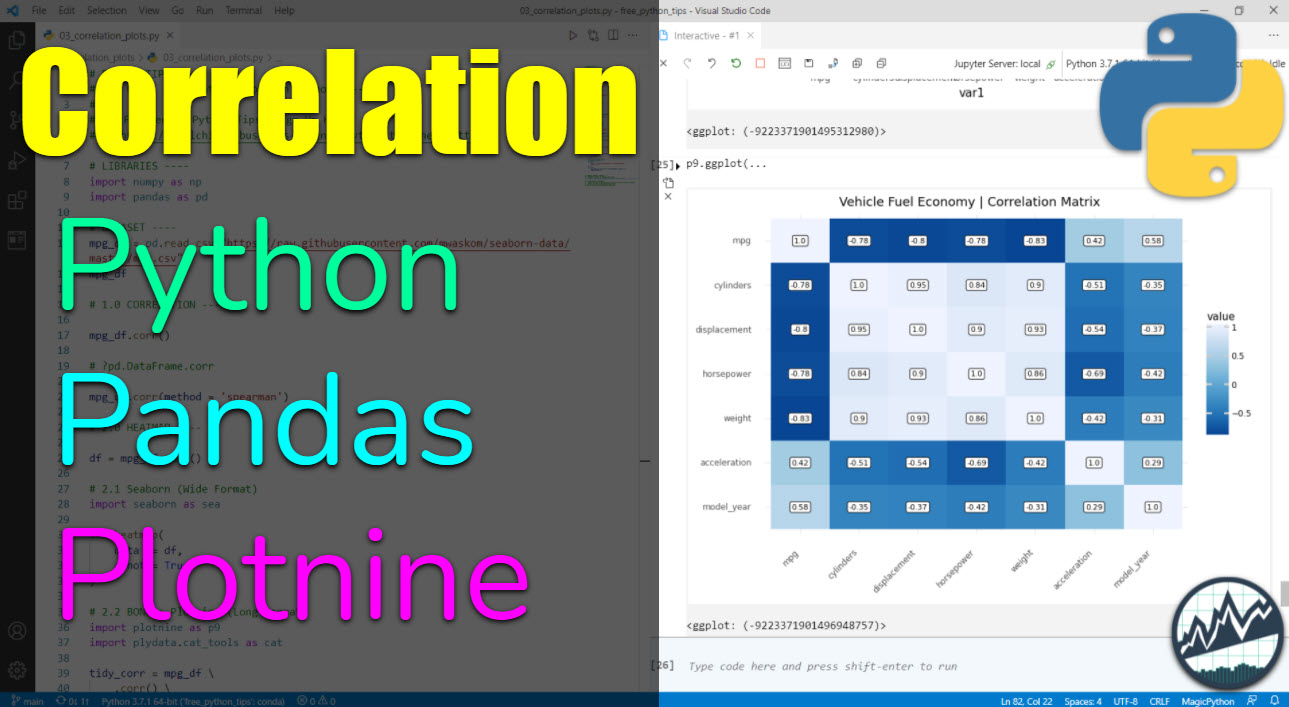### Hyperparameter Tuning Forecasts in Parallel with Modeltime

June 16, 2021 |

Speed up forecasting with modeltime’s new built-in parallel processing. Fitting many time series models can be an expensive process. To help speed up computation, modeltime now includes parallel processing, which is support for high-performance computing by spreading the model fitting steps across multiple CPUs or clusters. Highlights We now ...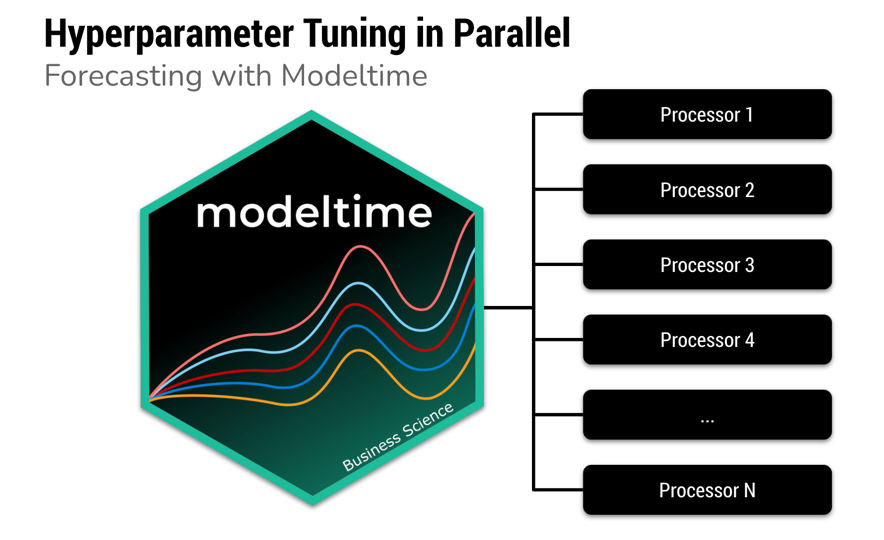### grafify: Make great-looking ggplot2 graphs quickly with R

June 14, 2021 |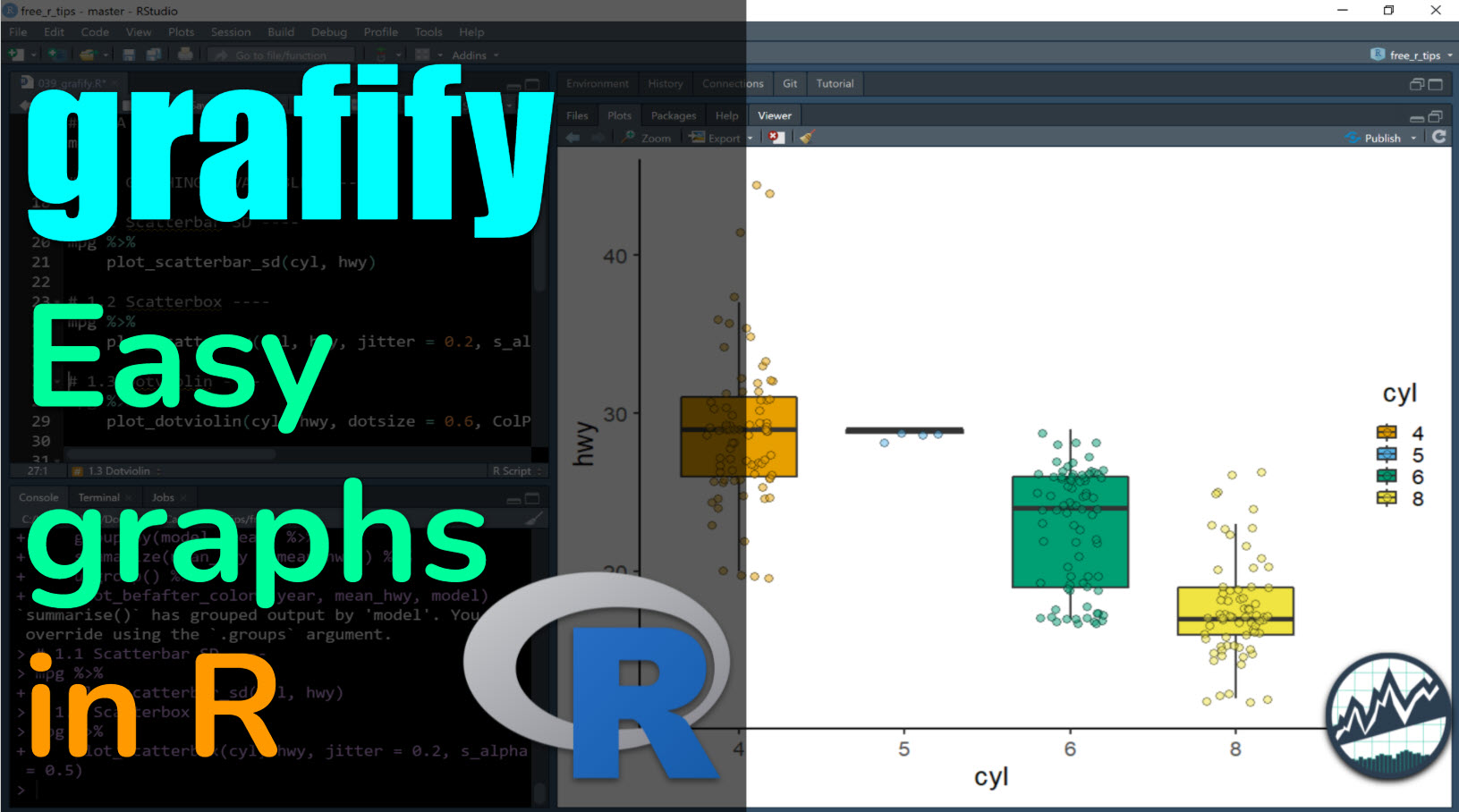### Siuba: Data wrangling with dplyr in Python

June 7, 2021 |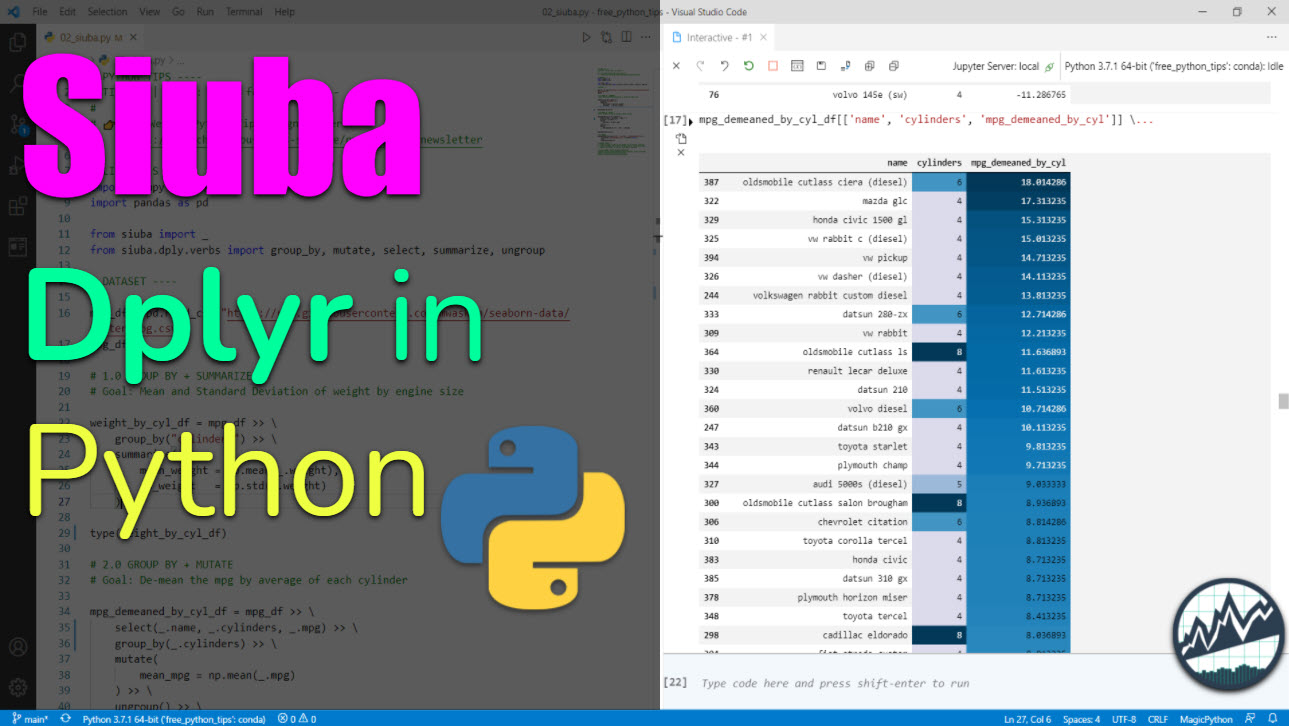### gghalves: Make Half Boxplot | Half Dotplot Visualizations with ggplot2

May 24, 2021 |

This article is part of R-Tips Weekly, a weekly video tutorial that shows you step-by-step how to do common R coding tasks. Here are the links to get set up. 👇 Get the Code YouTube Tutorial What is gghalves? gghalves is a new R package...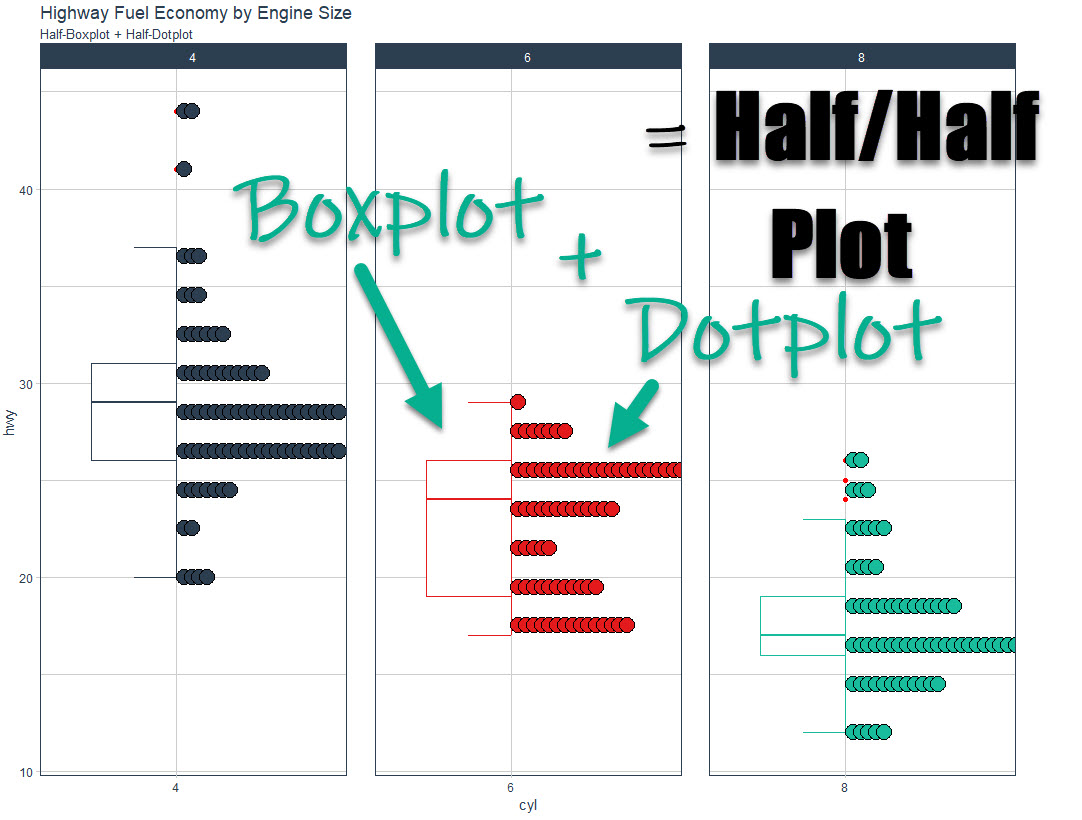### DataEditR: The GUI for Interactive Dataframe Editing in R

May 17, 2021 |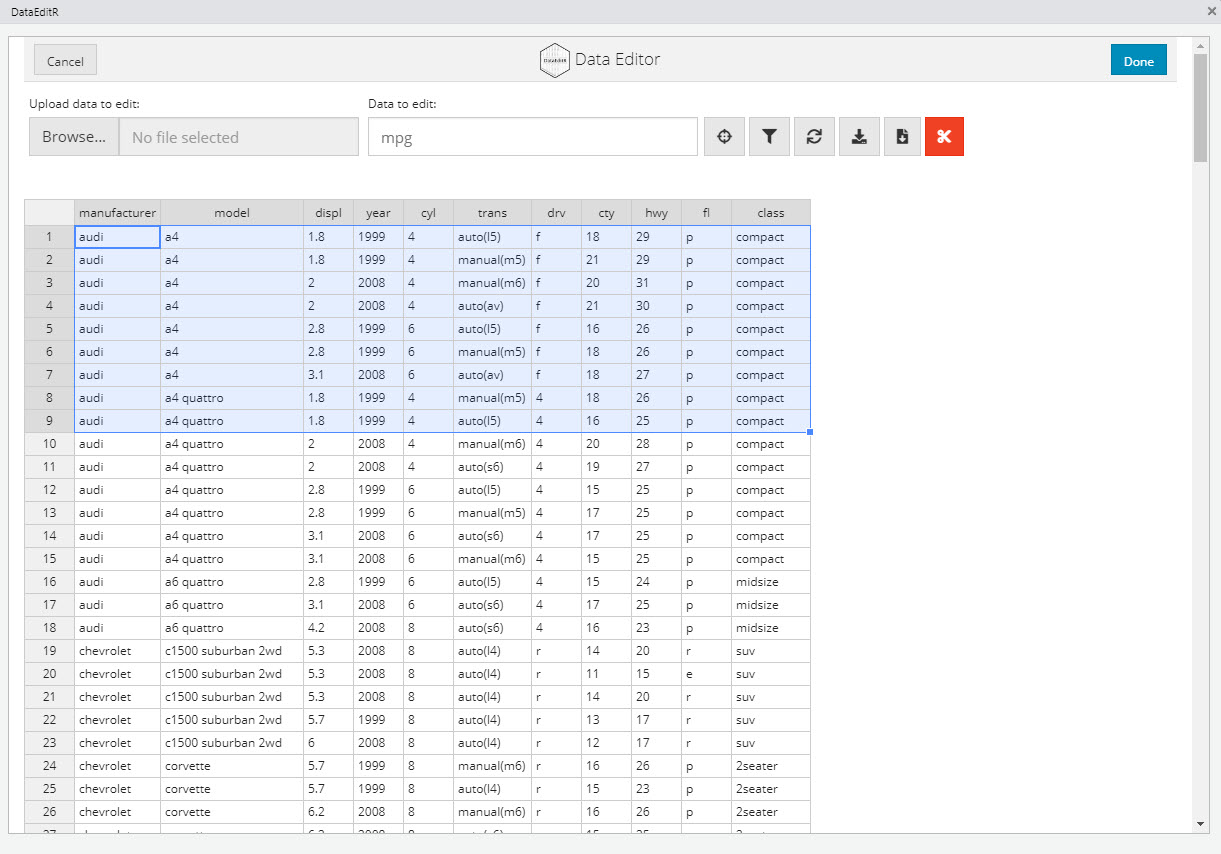### ggside: Plot Linear Regression using Marginal Distributions (ggplot2 extension)

May 17, 2021 |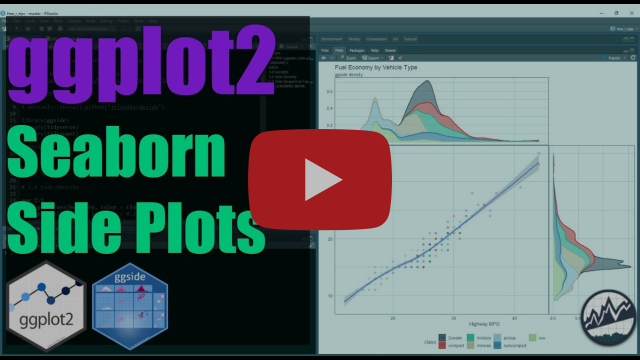### patchwork: ggplot2 plot combiner

May 10, 2021 |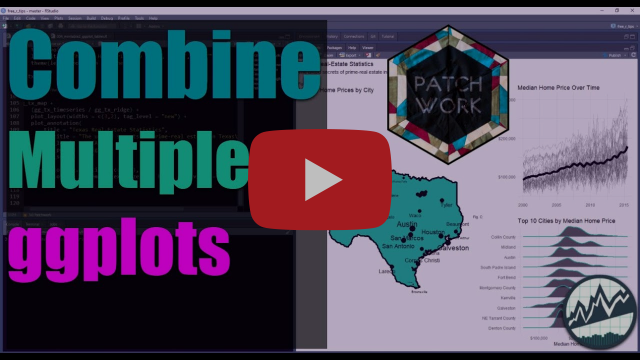### mmtable2: ggplot2 for tables

May 3, 2021 |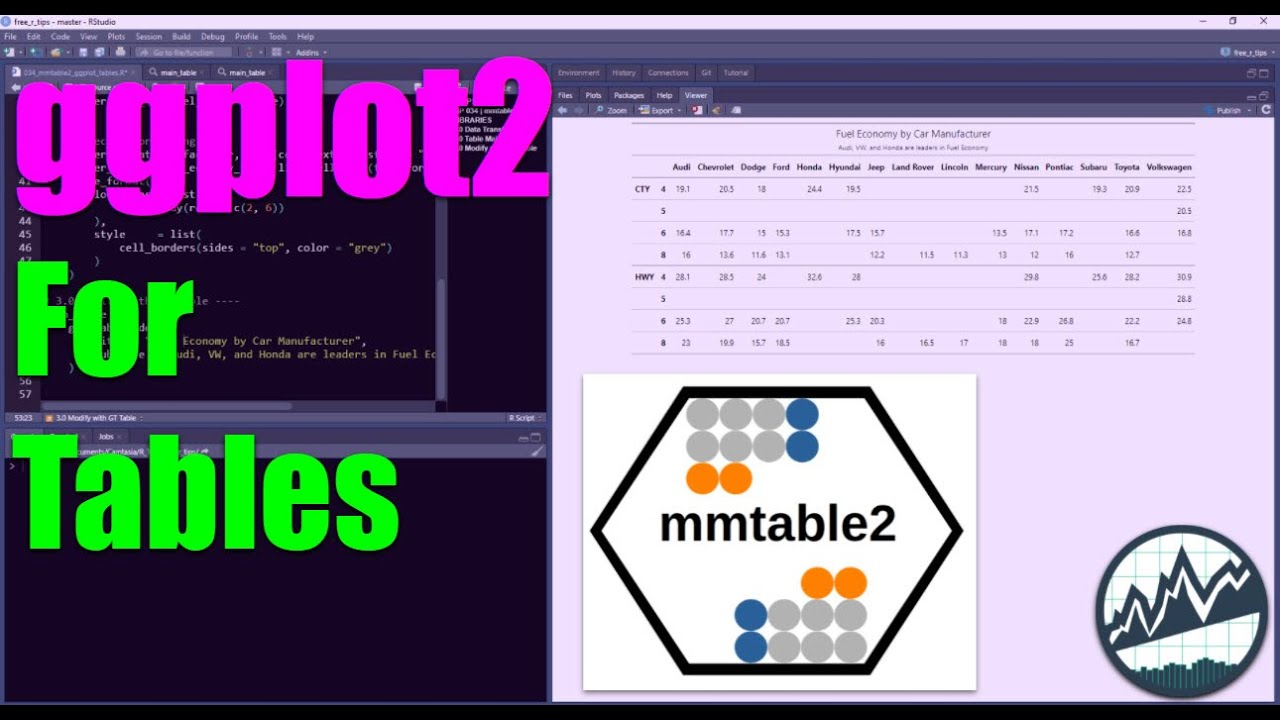### ggplot2 Extension: corrmorrant for Flexible Correlation Plots in R

April 26, 2021 |